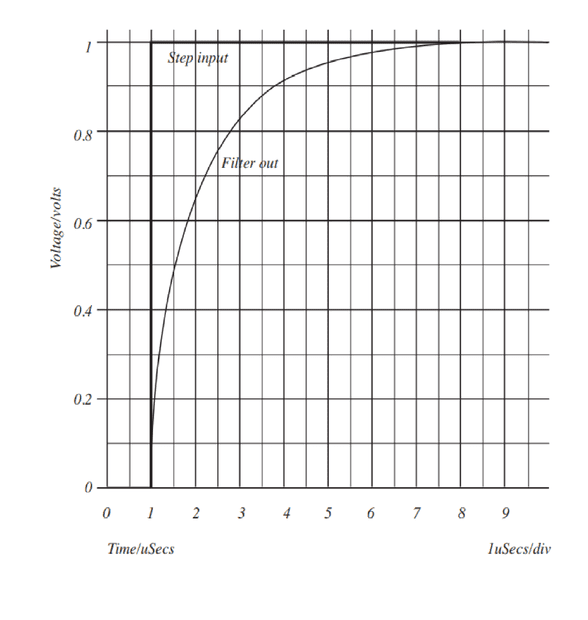# Half-power cut-off frequency and frequency and phase response.

• Engineering
• Callum Plunkett
In summary, a first-order filter has a half-power cut-off frequency of 127.27 kHz. It has a frequency response and phase response that is not easily Sketchable.f

#### Callum Plunkett

Homework Statement
Estimate the half-power cut-off frequency of the filter and sketch its
frequency and phase response.
Relevant Equations
Equation used if i am correct.
Tr = 0.35/Bandwidth
I have been given the attached following question:

FIGURE 2 shows the response of a first-order filter to a step input. Estimate the half-power cut-off frequency of the filter and sketch its frequency and phase response.

I believe that I have correctly calculated the half-power cut-off frequency as 127.27Khz. However, I am not sure how to begin sketch the frequency and phase response.However, I am not sure how to begin sketch the frequency and phase response.

What would a simple RC lowpass filter look like to generate this time domain response?

If you sketch that RC lowpass filter and write the transfer function ##\frac{V_o}{V_i}## using complex impedances, can you then plot the frequency domain magnitude and phase response?

•DaveE
•Averagesupernova, DaveE and berkeman
How did you arrive at f=127,27 kHz?

How did you arrive at f=127,27 kHz?
Yeah, I get slightly higher than that, based on the graph and his Relevant Equation...

•osilmag
Yeah, I get slightly higher than that, based on the graph and his Relevant Equation...
OK, but what about τ=RC=1/2πfg with τ=1µs at v(t)=0.63*Vmax?
Both results differ considerably. I suppose, the graph is not correct.

OK, but what about τ=RC=1/2πfg with τ=1µs at v(t)=0.63*Vmax?
Both results differ considerably. I suppose, the graph is not correct.
If folks were nitpicky enough, you could find a lot of disputes in textbooks.

Both results differ considerably. I suppose, the graph is not correct.
I believe the graph was given as part of the problem statement, no? And I think the OP was asked to calculate ##\tau## from the graph, so when the rising waveform makes it to ##1 - \frac{1}{e}##

I believe the graph was given as part of the problem statement, no? And I think the OP was asked to calculate ##\tau## from the graph, so when the rising waveform makes it to ##1 - \frac{1}{e}##
Yes - but there are two basic methods to find the 3dB-cut-off from the 1st-order step response :
a) based on the rise time (10% to 90%)
b) based on the 63% criterion (identical to the slope criterion for t=0).

The problem ist that the results differ considerably.
Therefore, I assume that the shown graph is not the correct step response for a 1st-order system.
(For example, the curve crosses the maximum voltage at 8µsec.)

•berkeman
Yes - but there are two basic methods to find the 3dB-cut-off from the 1st-order step response :
a) based on the rise time (10% to 90%)
b) based on the 63% criterion (identical to the slope criterion for t=0).

The problem ist that the results differ considerably.
Therefore, I assume that the shown graph is not the correct step response for a 1st-order system.
(For example, the curve crosses the maximum voltage at 8µsec.)
In my experience, practicing EEs always use the 10-90% definition of rise time, unless they've said otherwise. Physicists and mathematicians do whichever they like.

Yeah, good points by both of you. I guess we need to figure out where that rule of thumb of ##\frac{0.35}{t_R}## comes from, and which standard for rise time they used

Yeah, good points by both of you. I guess we need to figure out where that rule of thumb of ##\frac{0.35}{t_R}## comes from, and which standard for rise time they used
It's from 10-90% step response of a single pole.

It's from 10-90% step response of a single pole.
Link? (I'm in a Teams meeting so have not been able to do the search that I wanted...). Thanks Dave.

Or math. It also comes from that.

a single pole unit step response is ##f(t) = 1-e^{-\frac{t}{\tau}}## Solve for t gives ##t=- \tau \ln{(1-f(t))}##

So the 10-90% rise time ##t_r=- \tau (\ln{(1-0.9)} - \ln{(1-0.1)}) = \tau \ln{(9)} = 2.20 \tau##

But ##\tau = \frac{1}{2 \pi f_o}## so ##f_o = \frac{ln{(9)}}{2 \pi t_r} = \frac{0.35}{t_r}##

Last edited:
•Delta2 and berkeman
Note that one reason EE's tend to the 10-90% definition is that there are sometimes artifacts of other non-dominant poles/zeros or instrumentation issues that mess up the beginning and/or end of the step response. In the middle of the action, it is really the dominant stuff that shows up, then you can more easily ignore the noise, time delays, really fast responses, or slower perturbations.

•berkeman
Sorry for my very late reply, especially as I was looking for help.

The method I used was:
0.35/tr with when tr is 90-10 of the final value.

Thanks every one for the help provided and once again I apologise for the late reply.